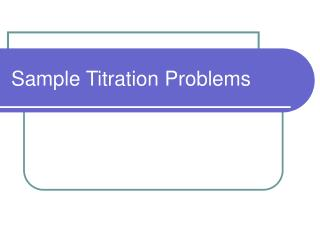DownloadDownload PresentationSample Titration Problems

# Sample Titration Problems

Télécharger la présentation## Sample Titration Problems

- - - - - - - - - - - - - - - - - - - - - - - - - - - E N D - - - - - - - - - - - - - - - - - - - - - - - - - - -
##### Presentation Transcript

1. Sample Titration Problems

2. Problem #1 A 20.00 mL sample of an unknown H3PO4 solution is titrated with a 0.100 M NaOH solution. The equivalence point is reached when 18.45 mL of NaOH solution is added. What is the concentration of the original H3PO4 solution?

3. H3PO4 (aq) + 3 NaOH (aq) 3 H2O (l) + Na3PO4 (aq)

4. Problem #1 0.100 moles NaOH* 0.01845 L NaOH = 0.001845 moles NaOH L NaOH solution 0.001845 moles NaOH * 1 mol OH-*1 mol H+ = 0.001845 mol H+ 1 mol NaOH1 mol OH- 0.001845 mol H+ * 1 mol H3PO4 = 0.0006150 mol H3PO4 3 mol H+ 0.0006150 mol H3PO4 = 0.03075 M H3PO4 0.020 L

5. Problem #1 i2M1V1 = i1M2V2 iNaOHMH3PO4VH3PO4 = iH3PO4 MNaOHVNaOH H3PO4 (aq) + 3 NaOH (aq) 3 H2O (l) + Na3PO4 (aq) (3) (MH3PO4) (20 mL) = (1) (0.100 M)(18.45 mL) MH3PO4 = 0.03075 M H3PO4

6. Problem #3 100.00 mL of a wastewater solution is diluted to 250.00 mL and titrated with 0.1106 M NaOH. Equivalence is reached after addition of 9.62 mL of the sodium hydroxide solution. What is the pH of the original wastewater sample?

7. H+ + OH- H2O i2M1V1 = i1M2V2 iOHMH+VH+ = iH+ MOHVOH (1) (MH+) (100 mL) = (1) (0.1106 M)(9.62 mL) MH+ = 0.01064 M H+ pH = - log [H+] = - log (0.01064 M) pH = 2.97

8. Problem #3 100.00 mL of wastewater is titrated with a 0.1062 M solution of Pb(NO3)2. Lead will react with chloride ion to form lead chloride. If it requires 6.52 mL of lead nitrate to reach equivalence, what was the concentration of chlorie in the original solution?

9. Pb(NO3)2 (aq) + 2 Cl-(aq) PbCl2 (s) + 2 NO3-(aq) Pb2+(aq)+ 2 Cl-(aq) PbCl2 (s) NO3- is a “spectator ion”

10. Pb2+(aq)+ 2 Cl-(aq) PbCl2 (s) i2M1V1 = i1M2V2 iCl-MPb2+VPb2+ = iPb+ MCl-VCl- (2) (0.1062 M) (6.52 mL) = (1) (MCl-)(100.00 mL) MCl- = 0.01385 M Cl-

11. Problem #4 A solution is prepared by mixing 0.10 L of 0.12 M sodium chloride with 0.23 L of a 0.18 M MgCl2 solution. What volume of a 0.20 M AgNO3 solution is required to precipitate all of the chloride ion as silver chloride?

12. Cl- (aq) + Ag+ (aq) AgCl(s) i2M1V1 = i1M2V2 iCl-MAg+VAg+ = iAg+ MCl-VCl- (1) (0.20 M) (VAg+) = (1) (MCl-)(VCl-) What is the volume of the chloride solution? What is the Molarity of Chloride?

13. (1) (0.20 M) (VAg+) = (1) (MCl-)(VCl-) What is the volume of the chloride solution? 0.10 L + 0.23 L = 0.33 L What is the Molarity of Chloride? (.12 M * 0.10 L) + (0.18M*0.23L) = 0.0534 mol Cl- 0.0534 mol Cl-= 0.1618 M Cl- 0.33 L

14. (1) (0.20 M) (VAg+) = (1) (MCl-)(VCl-) (1) (0.20 M) (VAg+) = (1) (0.1618 M-)(0.33 L) VAg+= 0.2670 L

15. Might have been easier just to go with the moles from beginning. Total moles of Cl- = 0.0534 mol Cl- [(.12 M * 0.10 L) + (0.18M*0.23L) = 0.0534 mol Cl-] 0.0534 mol Cl- * 1 mol Ag+ = 0.0534 mol Ag+ 1 mol Cl- 0.0534 mol Ag+ * 1 L Ag solution = 0.2670 L Ag solution 0.20 mol Ag solution